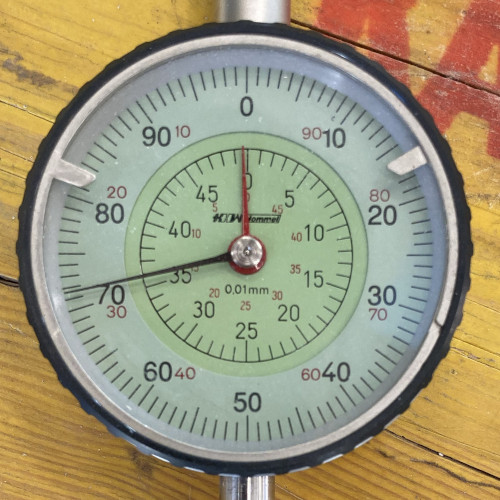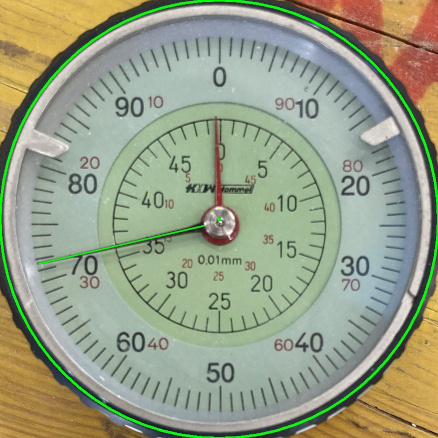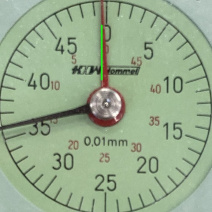# Python coding

## Read pointer-type dials on an analogue gauge with OpenCV

last updated: 2020-12-06

### Intro

We get the following picture and try to read the two pointers with python 3 and opencv.Being a newbie with opencv a good starting point and help for this code was the page of Nayak Abhijeet.

Here we see the result of our program:``````    gauge_p2.jpg    We are reading:  497.1153 µm
``````

### Installing python 3 and the needed modules

On Linux python3 is already installed. We need the following modules (libraries):

``````#!/usr/bin/python3
# -*- coding: utf-8 -*-

import os
import glob
import cv2 as cv      # to run the code even if version changes
import numpy as np
import pandas as pd
...
``````
• `os` is needed for operating system dependent functionality like change directory ('os.chdir(DIRNAMEIMAGES)')
• `glob2` finds all the pathnames matching a specified pattern and is used to get all files from an image directory.
• `cv2` is the opencv module to manipulate image data.
• `numpy` (NumPy) is the fundamental package for scientific computing in Python. It provides a multidimensional array object, and an assortment of routines for fast operations on arrays. Our images are such arrays.
• `NumPy` ()Pandas) provides a fast and efficient DataFrame object for data manipulation with integrated indexing. Pandas is top to work with spreadsheet data. We use pandas e.g. to create a `.csv`-file with the data.

On Linux use e.g `sudo apt install python3-opencv` to install the libraries, or use pip:

``````    pip3 install opencv-python
pip3 install pandas
``````

We use Python version 3. On Linux use always `python3` (not python or python2) to call python, also use `pip3` instead of pip.

``````    python3 read_analogue_gauge.py
``````

To work on the program you can use Idle, Python’s Integrated Development Environment, but we prefer the Thonny IDE.

To run the program you need to adapt the directory path for the image files:

``````...
...
``````
##### for Windows user:

If you can't change to Linux and are forced to use Windows :)).

Download python on python.org and install the `.exe` file. On the first screen check "Add Python to PATH". This enables you to use python everywhere.

Open command prompt (Start and type `cmd`) and use the following commands to install the missing modules:

``````    pip install opencv-python
pip install pandas
``````

To work on the program you can use `IDLE`, Python’s Integrated Development Environment, that was already installed with the `.exe`-file. We prefer the Thonny IDE, that also exists for Windows.

In Windows we need to adapt the directory path. The backslash needs to get doubled, like this:

``````...
...
``````

If further help for python on Windows is needed click here: Using Python on Windows.

### Opencv methods

An overview on OpenCV methods can be found on my OpenCV basics page:

http://weigu.lu/other_projects/python_coding/opencv_basics/index.html

That is a good starting point to understand the following code.

### The python code

``````    #!/usr/bin/python3
# -*- coding: utf-8 -*-

""" Read pointer-type dials on an analogue gauge """
###############################################################################
#
#  Purpose:      read pointer-type dials on an analogue gauge
#  Author:       weigu.lu
#  Date:         2020-12-06
#  Version       1.1
#
#
#  This program is free software; you can redistribute it and/or modify
#  the Free Software Foundation; either version 2 of the License, or
#  (at your option) any later version.
#
#  This program is distributed in the hope that it will be useful,
#  but WITHOUT ANY WARRANTY; without even the implied warranty of
#  MERCHANTABILITY or FITNESS FOR A PARTICULAR PURPOSE.  See the
#  GNU General Public License for more details.
#
#  You should have received a copy of the GNU General Public License
#  along with this program; if not, write to the Free Software
#  Foundation, Inc., 51 Franklin Street, Fifth Floor, Boston,
#  MA 02110-1301, USA.
#
#  source:
#  https://medium.com/@nayak.abhijeet1/
#
#
###############################################################################

import os
import glob
import cv2 as cv                        # to run code even if version changes
import numpy as np
import pandas as pd

MAX_PIXEL = 1000                        # reduce picture to this size for quicker calculations

DEBUG = 0

MIN_RADIUS_RATIO_1 = 0.8                # Where to look for the first circle
MIN_RADIUS_RATIO_2 = 0.85               # Where to look for the second circle

L1_P1_LOW = 0                           # boundary how close the line (P1) should be from the center
L1_P1_UP = 0.3
L1_P2_LOW = 0.7                         # how close P2 should be to the outside of the gauge
L1_P2_UP = 0.9

L2_P1_LOW = 0                           # boundary how close the line (P1) should be from the center
L2_P1_UP = 0.3
L2_P2_LOW = 0.8                         # how close P2 should be to the outside of the gauge
L2_P2_UP = 0.995

### the functions ###

def avg_circles(pcircles, pb):
'''averaging out nearby circles'''
avg_x = 0
avg_y = 0
avg_r = 0
for i in range(pb):                 # optional: av. multiple circles (gauge at a slight angle)
avg_x = avg_x + pcircles[i]
avg_y = avg_y + pcircles[i]
avg_r = avg_r + pcircles[i]
avg_x = int(avg_x/(pb))
avg_y = int(avg_y/(pb))
avg_r = int(avg_r/(pb))
return avg_x, avg_y, avg_r

def dist_2_pts(px1, py1, px2, py2):
'''pythagore'''
return np.sqrt((px2 - px1)**2 + (py2 - py1)**2)

def show_image(pimg, show_flag_time):
''' Show image during x ms if flag is set. Parameter show_flag_time is a
tuple e.g (1,2000) to show picture for 2s or (0,2000) to prevent the
show. (1,0) waits on keypress'''
if show_flag_time == 1:
cv.imshow('image', pimg)        # cv.imshow(window_name, image)
cv.waitKey(show_flag_time)   # show picture for x ms (x=0 for keypress)
cv.destroyAllWindows()

def get_img_reduce_size(l_img_name, max_pixel):
'''get image and reduce size to max_pixel'''
if DEBUG:
print('Original image shape: ', mimg.shape)
row, col = mimg.shape[:2]           # get number of rows (height), columns (width)
if row >= col and row > max_pixel:  # calculate ratio to reduce image
ratio = max_pixel/row
elif col >= row and col > max_pixel:
ratio = max_pixel/col
else:
ratio = 1.0
mimg = cv.resize(mimg, (0, 0), fx=ratio, fy=ratio)
mheight, mwidth = mimg.shape[:2]
if DEBUG:
print('Reduced image shape: ', mheight, mwidth)
mgrey_img = cv.cvtColor(mimg, cv.COLOR_BGR2GRAY) # convert to grey image
return mimg, mgrey_img, mheight, mwidth

def get_circle_and_crop_image(pimg, red_ratio, minrr, maxrr):
''' Reduce image size with red_ratio (needed for second pointer
Getting circles using HoughCircles. Important for a good result are the
mheight, mwidth = pimg.shape[:2]
new_height = int(mheight*red_ratio)
new_width = int(mwidth*red_ratio)
mx1 = (mwidth - new_width)
my1 = (mheight - new_height)
mimg = pimg[my1:new_height, mx1:new_width]
mgrey_img = cv.cvtColor(mimg, cv.COLOR_BGR2GRAY) # convert to grey image
mgrey_blured_img = cv.medianBlur(mgrey_img, 5)
mheight, mwidth = mgrey_blured_img.shape[:2]
circles = cv.HoughCircles(mgrey_blured_img, cv.HOUGH_GRADIENT, 1, 20, np.array([]), 100, 50,
int(mheight*minrr/2), int(mheight*maxrr/2))
b = circles.shape
if DEBUG:
print('Number of circles: ', b)
mcircles_img = mimg.copy()
mcircle_img = mimg.copy()
for (mx, my, mr) in circles[0, :]:
cv.circle(mcircles_img, (int(mx), int(my)), int(mr), (0, 255, 0), 3)
cv.circle(mcircles_img, (int(mx), int(my)), 2, (0, 255, 0), 3)
mx, my, mr = avg_circles(circles, b) # averaging out nearby circles
cv.circle(mcircle_img, (mx, my), mr, (0, 255, 0), 3)
cv.circle(mcircle_img, (mx, my), 2, (0, 255, 0), 3)
rect_x = (mx - mr)                  # crop image to circle (x=r, y=r)
rect_y = (my - mr)
cropped_img = mimg[rect_y:(rect_y+2*mr), rect_x:(rect_x+2*mr)]
cropped_circle_img = mcircle_img[rect_y:(rect_y+2*mr), rect_x:(rect_x+2*mr)]
cropped_grey_img = mgrey_img[rect_y:(rect_y+2*mr), rect_x:(rect_x+2*mr)]
mheight, mwidth = cropped_circle_img.shape[:2]
if DEBUG:
print('Reduced image shape: ', mheight, mwidth)
return mr, mr, mr, mcircles_img, cropped_img, cropped_circle_img, \
cropped_grey_img

def get_pointer(px, py, pimg, pgrey_img, p1_b_low, p1_b_up, p2_b_low, p2_b_up):
''' Create a threshhold image to get lines using HoughLinesP. '''
THRESH = 100                        # test which threshhold function performs best
MAX_VALUE = 180
MIN_LINE_LENGTH = 100
MAX_LINE_GAP = 10
mgrey_img = cv.medianBlur(pgrey_img, 5)
threshhold_img = cv.threshold(mgrey_img, THRESH, MAX_VALUE, cv.THRESH_BINARY_INV)
lines = cv.HoughLinesP(image=threshhold_img, rho=3, theta=np.pi / 180, threshold=100,
minLineLength=MIN_LINE_LENGTH, maxLineGap=MAX_LINE_GAP)
mlines_img = pimg.copy()
mline_img = pimg.copy()
for line in lines:                  # create image with lines
mx1, my1, mx2, my2 = line
cv.line(mlines_img, (mx1, my1), (mx2, my2), (0, 255, 0), 2)
p1_b_low = 0                        # how close the line (P1) should be from the center
p1_b_up = 0.3
p2_b_low = 0.7                      # how close P2 should be to the outside of the gauge
p2_b_up = 0.9
mx1, my1, mx2, my2 = calculate_pointer(px, py, lines, p1_b_low, p1_b_up,
p2_b_low, p2_b_up)
cv.line(mline_img, (mx1, my1), (mx2, my2), (0, 255, 0), 2) # create image w line
return mx1, my1, mx2, my2, threshhold_img, mlines_img, mline_img

def calculate_pointer(px, py, plines, p1_b_low, p1_b_up, p2_b_low, p2_b_up):
'''calculate the pointer'''
final_line_list = []
for i, pline in enumerate(plines):
for mx1, my1, mx2, my2 in pline:
diff1 = dist_2_pts(px, py, mx1, my1)  # x, y is center of circle
diff2 = dist_2_pts(px, py, mx2, my2)  # x, y is center of circle
if diff1 > diff2:             # set diff1 to be the smaller (closest to center)
diff1, diff2 = diff2, diff1  # of the two,makes the math easier
if (p1_b_low*r < diff1 < p1_b_up*r) and \
(p2_b_low*r < diff2 < p2_b_up*r): # check if in acceptable range
final_line_list.append([mx1, my1, mx2, my2]) # add to final list
try:
mx1 = final_line_list
my1 = final_line_list
mx2 = final_line_list
my2 = final_line_list
except IndexError:
print("\nWrong matching line found? recheck this part of code!\n")
return mx1, my1, mx2, my2

def get_red(pimg):
'''filter the red channel'''
img_hsv = cv.cvtColor(pimg, cv.COLOR_BGR2HSV)
red_min = np.array([0, 60, 0])
red_max = np.array([10, 255, 255])
return ~mask                          # return inverted image

def calculate_angle_and_value(px, py, px1, py1, px2, py2):
'''calculate the angle and value'''
dist_pt0 = dist_2_pts(px, py, px1, py1)
dist_pt1 = dist_2_pts(px, py, px2, py2)
if dist_pt0 > dist_pt1:
xlen = px1 - px
ylen = py - py1
else:
xlen = px2 - px
ylen = py - py2
if xlen == 0:
xlen = 0.0000000000000000001
res = np.arctan(np.divide(float(abs(ylen)), float(abs(xlen)))) # arc-tan
if DEBUG:
print("res", res)
print("xlen, ylen", xlen, ylen)
if xlen < 0 and ylen > 0:             # quadrant 4
final_angle = res + 270
if xlen > 0 and ylen > 0:             # quadrant 1
final_angle = 90 - res
if xlen > 0 and ylen < 0:             # quadrant 2
final_angle = 90 + res
if xlen < 0 and ylen < 0:             # quadrant 3
final_angle = 270 - res
value = final_angle/360*100
return final_angle, value

# flags and times in ms to show images
flag = {"reduced":(0, 500), "grey":(0, 500), "circles":(0, 500),
"circle":(0, 500), "threshhold":(0, 500), "lines":(0, 500),
"line":(1, 500), "circles_2":(0, 500), "circle_2":(0, 1500),
"grey_2":(0, 5000), "threshhold_2":(0, 200), "lines_2":(0, 500),
"line_2":(1, 500)}

os.chdir(DIR_NAME_IMAGES)                 # change directory
img_list = glob.glob('*.jpg')             # get list with jpg images
img_list.extend(glob.glob('*.png'))       # and png images
img_list.sort()
if img_list == []:
print("error: no images!")
data_saved = dict.fromkeys(img_list, 0)   # create directory from list
print(data_saved)

for img_name in img_list:
print(img_name, end='')
img, grey_img, height, width = get_img_reduce_size(img_name, MAX_PIXEL)
show_image(img, flag["reduced"])
x, y, r, circles_img, img, circle_img, grey_img = \
show_image(grey_img, flag["grey"])
show_image(circles_img, flag["circles"])
show_image(circle_img, flag["circle"])
x1, y1, x2, y2, threshhold_image, lines_img, line_img = \
get_pointer(r, r, circle_img, grey_img, L1_P1_LOW, L1_P1_UP, L1_P2_LOW,
L1_P2_UP)
show_image(threshhold_image, flag["threshhold"])
show_image(lines_img, flag["lines"])
show_image(line_img, flag["line"])
# cv.imwrite("first_pointer.jpg",line_img)
final_angle_1, value_1 = calculate_angle_and_value(x, y, x1, y1, x2, y2)
x, y, r, circles_img, img, circle_img, grey_img = \
show_image(circles_img, flag["circles_2"])
show_image(circle_img, flag["circle_2"])
grey_img = get_red(circle_img)        # to eliminate black pointer
show_image(grey_img, flag["grey_2"])
show_image(grey_img, flag["circle_2"])
x1, y1, x2, y2, threshhold_image, lines_img, line_img = \
get_pointer(r, r, img, grey_img, L2_P1_LOW, L2_P1_UP, L2_P2_LOW,
L2_P2_UP)
show_image(threshhold_image, flag["threshhold_2"])
show_image(lines_img, flag["lines_2"])
show_image(line_img, flag["line_2"])
# cv.imwrite("second_pointer.jpg",line_img)
final_angle_2, value_2 = calculate_angle_and_value(x, y, x1, y1, x2, y2)
if DEBUG:
print(final_angle_1, value_1)
print(final_angle_2, value_2)
result = round((int(value_2/2) + value_1/100)*10, 4)
print("\tWe are reading: ", result, "µm\n")
data_saved[img_name] = result

if DEBUG:
print(data_saved)
df = pd.DataFrame(data_saved, index=)  # pandas dataframe
df.to_csv("my_data.csv", index=False)     # save data to csv file
``````